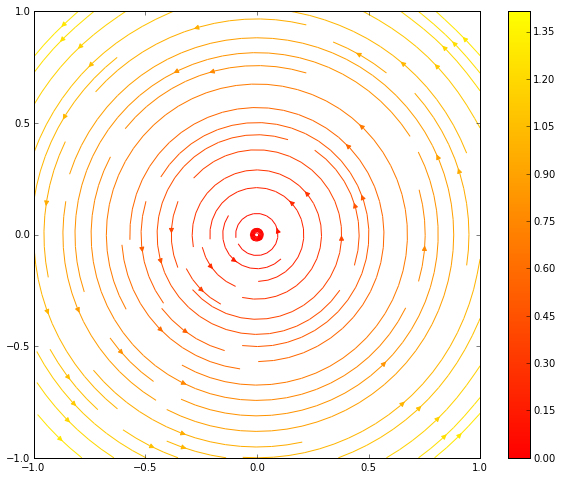# Differential Equation Notes

## Reference for odeint

We saw that Scipy provides the odeint interface to numerically integrating ODEs. As a reference for how this works, here's an example in 1D and for a system:

In :
%matplotlib inline
import numpy as np
import matplotlib.pyplot as plt
from scipy.integrate import odeint

def f(x, t):
return x * (1 - x)

t = np.linspace(0, 5, 100) # <- time slices to compute
x0 = 0.1                   # <- initial condition
x = odeint(f, x0, t)       # <- solve ODE

plt.figure()
plt.plot(t, x)
plt.show()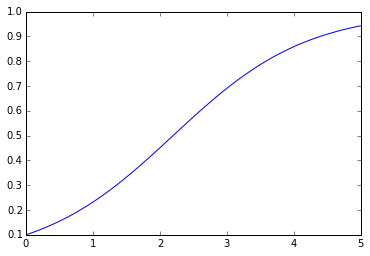The only change required for a system of ODEs is that we need to provide odeint with an array instead of a scalar.

In :
%matplotlib inline
import numpy as np
import matplotlib.pyplot as plt
from scipy.integrate import odeint

def f(state, t):
x, y = state           # <- now odeint gives us an array of data
return np.array([      # <- we'll return an array representing the vector field at the point
-y,
x,
])

t = np.linspace(0, 8, 100) # <- time slices to compute
x0 = np.array([1.0, 1.0])  # <- initial condition (now an array!)
soln = odeint(f, x0, t)    # <- solve ODE

plt.figure()
plt.plot(soln[:, 0], soln[:, 1])
plt.show()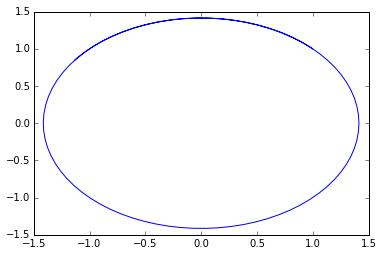## Quiver and Streamplots

We saw some streamplot examples. I just wanted to give a reference to how to use these here. We didn't actually discuss quiver, but the interface is essentially the same as streamplot.

In :
%matplotlib inline
import numpy as np
import matplotlib.pyplot as plt

x, y = np.meshgrid(np.linspace(-1, 1, 25),
np.linspace(-1, 1, 25))

u = -y
v = x

plt.figure(figsize=(8, 8))
plt.xlim(-1, 1)
plt.ylim(-1, 1)
plt.quiver(x, y, u, v)
plt.show()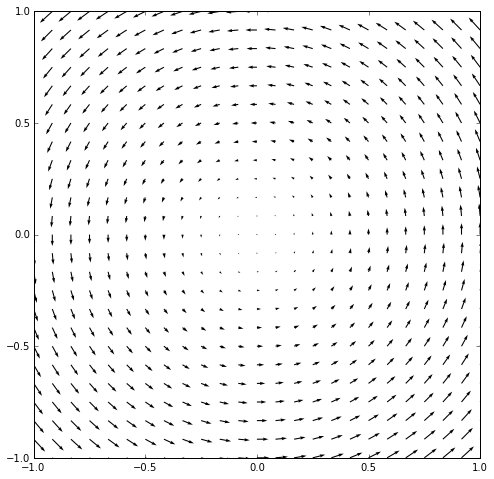In :
plt.figure(figsize=(8, 8))
plt.xlim(-1, 1)
plt.ylim(-1, 1)
plt.streamplot(x, y, u, v)
plt.show()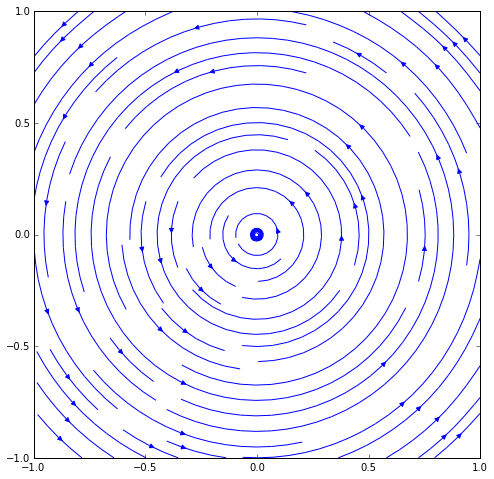I did mention that we can color the plot according to various quantities. For example, if we wanted to color by the magnitude of the vectors we can use:

In :
from numpy.linalg import norm

# dstack takes our 2D arrays of u, v values and combines
# them into a coherent 2D array of (u, v) pairs. I'm just
# using this to compute the norm of each vector.
mag = norm(np.dstack([u, v]), axis=-1)

plt.figure(figsize=(10, 8))
plt.xlim(-1, 1)
plt.ylim(-1, 1)
plt.streamplot(x, y, u, v, color=mag, cmap='autumn')
plt.colorbar()
plt.show()